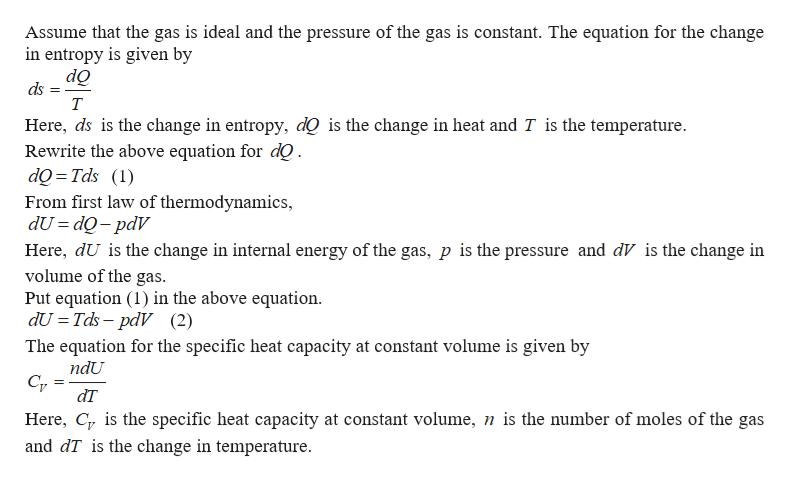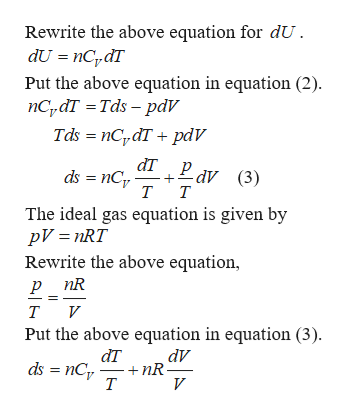# Derive a formula for the entropy of change in a gas that is absorbing heat causing it to go from TL to TH. Express in terms of specific heat of gas, number of moles, R, and TL, TH.

Question
3 views

Derive a formula for the entropy of change in a gas that is absorbing heat causing it to go from Tto TH. Express in terms of specific heat of gas, number of moles, R, and TL, TH.

check_circle

star
star
star
star
star
1 Rating
Step 1help_outlineImage TranscriptioncloseAssume that the gas is ideal and the pressure of the gas is constant. The equation for the change in entropy is given by do ds т Here, ds is the change in entropy, dQ is the change in heat and T is the temperature Rewrite the above equation for dQ dQ Tds () From first law of thermodynamics, dU dQ-pdV Here, dU is the change in internal energy of the gas, p is the pressure and dV is the change in volume of the gas. Put equation (1) in the above equation dU Tds-pdV (2) The equation for the specific heat capacity at constant volume is given by ndU dT Here, C is the specific heat capacity at constant volume, n is the number of moles of the gas and dT is the change in temperature fullscreen
Step 2help_outlineImage TranscriptioncloseRewrite the above equation for dU dU nCdT Put the above equation in equation (2) nCydT Tds - pdV Tds nCpdT pdV dT Pav ds nCT (3) т т The ideal gas equation is given by pV nRT Rewrite the above equation, р nR т и Put the above equation in equation (3) dт dV nR т ds nC V fullscreen

### Want to see the full answer?

See Solution

#### Want to see this answer and more?

Solutions are written by subject experts who are available 24/7. Questions are typically answered within 1 hour.*

See Solution
*Response times may vary by subject and question.
Tagged in

### Physics